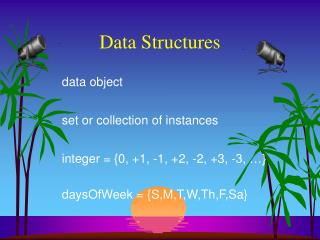# Data Structures - PowerPoint PPT PresentationDownload PresentationData Structures

Data StructuresDownload Presentation## Data Structures

- - - - - - - - - - - - - - - - - - - - - - - - - - - E N D - - - - - - - - - - - - - - - - - - - - - - - - - - -
##### Presentation Transcript

1. Data Structures data object set or collection of instances integer = {0, +1, -1, +2, -2, +3, -3, …} daysOfWeek = {S,M,T,W,Th,F,Sa}

2. Data Object instances may or may not be related myDataObject = {apple, chair, 2, 5.2, red, green, Jack}

3. Data Structure Data object + relationships that exist among instances and elements that comprise an instance Among instances of integer 369 < 370 280 + 4 = 284

4. Data Structure Among elements that comprise an instance 369 3 is more significant than 6 3 is immediately to the left of 6 9 is immediately to the right of 6

5. Data Structure The relationships are usually specified by specifying operations on one or more instances. add, subtract, predecessor, multiply

6. Linear (or Ordered) Lists instances are of the form (e0, e1, e2, …, en-1) where ei denotes a list element n >= 0 is finite list size isn

7. Linear Lists L = (e0, e1, e2, e3, …, en-1) relationships e0 is the zero’th (or front) element en-1 is the last element eiimmediately precedes ei+1

8. Linear List Examples Students in COP3530 = (Jack, Jill, Abe, Henry, Mary, …, Judy) Exams in COP3530 = (exam1, exam2, exam3) Days of Week = (S, M, T, W, R, F, Sa) Months = (Jan, Feb, Mar, Apr, …, Nov, Dec)

9. Linear List Operations—size() determine list size L = (a,b,c,d,e) size = 5

10. Linear List Operations—get(theIndex) get element with given index L =(a,b,c,d,e) get(0) = a get(2) = c get(4) = e get(-1) = error get(9) = error

11. Linear List Operations—indexOf(theElement) determine the index of an element L =(a,b,d,b,a) indexOf(d) = 2 indexOf(a) = 0 indexOf(z) = -1

12. Linear List Operations—remove(theIndex) remove and return element with given index L = (a,b,c,d,e,f,g) remove(2) returns c and L becomes (a,b,d,e,f,g) index of d,e,f, and g decrease by 1

13. Linear List Operations—remove(theIndex) remove and return element with given index L = (a,b,c,d,e,f,g) remove(-1) => error remove(20) => error

14. Linear List Operations—add(theIndex, theElement) add an element so that the new element has a specified index L = (a,b,c,d,e,f,g) add(0,h) => L = (h,a,b,c,d,e,f,g) index of a,b,c,d,e,f, and g increase by 1

15. Linear List Operations—add(theIndex, theElement) L = (a,b,c,d,e,f,g) add(2,h) => L = (a,b,h,c,d,e,f,g) index of c,d,e,f, and g increase by 1 add(10,h) => error add(-6,h) => error

16. Data Structure Specification • Language independent • Abstract Data Type • Java • Interface • Abstract Class

17. Linear List Abstract Data Type AbstractDataTypeLinearList { instances ordered finite collections of zero or more elements operations isEmpty(): return true iff the list is empty, falseotherwise size(): return the list size (i.e., number of elements in the list) get(index): return theindexth element of the list indexO f(x): return the index of the first occurrence of xin the list, return -1 ifxis not in the list remove(index): remove and return theindexth element, elements with higher index have their index reduced by 1 add(theIndex, x): insert x as the indexth element, elements with theIndex >= indexhave their index increased by1 output(): output the list elements from left to right }

18. Linear List as Java Interface An interface may include constants and abstract methods (i.e., methods for which no implementation is provided).

19. Linear List as Java Interface public interface LinearList { public boolean isEmpty(); public int size(); public Object get(int index); public int indexOf(Object elem); public Object remove(int index); public void add(int index, Object obj); public String toString(); }

20. Implementing An Interface public classArrayLinearList implementsLinearList { // code for all LinearList methods must be provided here }

21. Linear List As An Abstract Class An abstract class may include constants, variables, abstract methods, and nonabstract methods.

22. Linear List As Java Abstract Class publicabstract class LinearListAsAbstractClass { public abstract boolean isEmpty(); public abstract int size(); public abstract Object get(int index); public abstract int indexOf(Object theElement); public abstract Object remove(int index); public abstract void add(int index, Object theElement); public abstract String toString(); }

23. Extending A Java Class public class ArrayLinearList extends LinearListAsAbstractClass { // code for all abstract classes must come here }

24. Implementing Many Interfaces public classMyInteger implementsOperable, Zero, CloneableObject { // code for all methods of Operable, Zero, // and CloneableObject must be provided }

25. Extending Many Classes NOT PERMITTED IN JAVA A Java class may implement as many interfaces as it wants but can extend at most 1 class.

26. Data Structures In Text All but 1 of our data structures are specified as Java interfaces. Exception is Graph in Chapter 17 (abstract class) Java specifies all of its data structures as interfaces. java.util.List

27. Summary • ADT – language independent behavior • Interface – ADT + typing (constants, abstract methods) • Implementation – defines methods, etc. for all interfaces implemented • Abstract class – interface+nonabstract methods, variables • Extension – inherits superclass methods, must define abstract methods

28. Next – Lecture 5 • Data Representation Methods • Read rest of Chapter 5